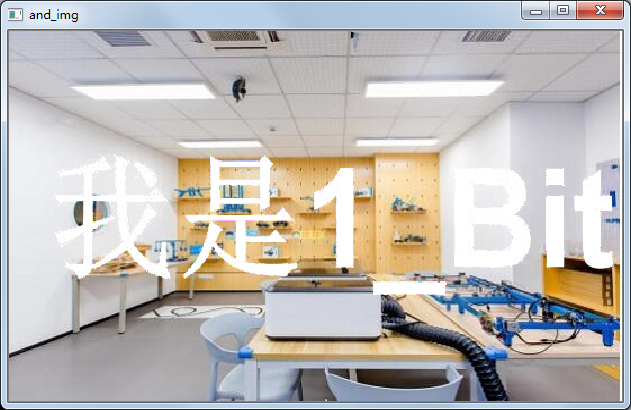# [python opencv 计算机视觉零基础到实战] 七、逻辑运算与应用1人已赏
1_bit 发布于 2020-12-28 18:56:56 浏览 2838 点赞 35 收藏 9

# 一、学习目标

1. 了解opencv中图像的逻辑运算
2. 了解opencv中逻辑运算的应用

# 二、了解OpenCV中图像运算的运用

## 2.1 了解and逻辑运算

``````import cv2

img1 = cv2.imread(r'C:\Users\mx\Desktop\1bit.jpg')
img3 = cv2.imread(r'C:\Users\mx\Desktop\1bit3.jpg')

cv2.imshow("img1", img1)
cv2.imshow("img3", img3)

cv2.waitKey (0)
cv2.destroyAllWindows()``````

``````import cv2

img1 = cv2.imread(r'C:\Users\mx\Desktop\1bit.jpg')
img3 = cv2.imread(r'C:\Users\mx\Desktop\1bit3.jpg')
and_img=cv2.bitwise_and(img3,img1)
cv2.namedWindow("and_img",cv2.WINDOW_NORMAL)

cv2.imshow("img1", img1)
cv2.imshow("img3", img3)
cv2.imshow("and_img", and_img)

cv2.waitKey (0)
cv2.destroyAllWindows()``````

## 2.2 了解or逻辑运算

``````import cv2

img1 = cv2.imread(r'C:\Users\mx\Desktop\1bit.jpg')
img3 = cv2.imread(r'C:\Users\mx\Desktop\1bit3.jpg')
and_img=cv2.bitwise_or(img3,img1)
cv2.namedWindow("and_img",cv2.WINDOW_NORMAL)

cv2.imshow("img1", img1)
cv2.imshow("img3", img3)
cv2.imshow("and_img", and_img)

cv2.waitKey (0)
cv2.destroyAllWindows()``````## 2.3 了解取反运算

``````import cv2

img1 = cv2.imread(r'C:\Users\mx\Desktop\1bit.jpg')
not_img=cv2.bitwise_not(img1)
cv2.imshow("img1 ", img1 )
cv2.imshow("not_img", not_img)
cv2.waitKey (0)
cv2.destroyAllWindows()``````

## 2.4 逻辑运算有什么用呢？

``````import cv2
import numpy as np

capture=cv2.VideoCapture(r'C:\Users\mx\Desktop\hmbb.mp4')
min=np.array([26,43,46])
max=np.array([34,255,255])
while True:
r,img=capture.read()
if r==False:
break
hsv_img=cv2.cvtColor(img,cv2.COLOR_BGR2HSV)
flag=cv2.inRange(hsv_img,lowerb=min,upperb=max)
cv2.imshow("flag", flag)
cv2.imshow("hmbb", img)
k=cv2.waitKey (40)
if k==27:
break
cv2.destroyAllWindows()``````

``cflag=cv2.bitwise_and(img,img,mask=flag)``

``````import cv2
import numpy as np

capture=cv2.VideoCapture(r'C:\Users\mx\Desktop\hmbb.mp4')
img1 = cv2.imread(r'C:\Users\mx\Desktop\1bit.jpg')
min=np.array([26,43,46])
max=np.array([34,255,255])
while True:
r,img=capture.read()
if r==False:
break
hsv_img=cv2.cvtColor(img,cv2.COLOR_BGR2HSV)
flag=cv2.inRange(hsv_img,lowerb=min,upperb=max)
cflag=cv2.bitwise_and(img,img,mask=flag)
cv2.imshow("flag", flag)
cv2.imshow("hmbb", img)
cv2.imshow("cflag", cflag)
k=cv2.waitKey (40)
if k==27:
break
cv2.destroyAllWindows()``````

# 三、总结

1. 了解了opencv中对图像进行逻辑与运算使用bitwise_and方法
2. 了解了opencv中对图像进行逻辑或运算使用bitwise_or方法
3. 了解了opencv中对图像进行取反运算使用bitwise_not方法
4. 了解了and方法的其他用途，用于mask遮罩剔除不需要的内容
CSDN博客专家，科技博主，十多年开发经验。

*本文仅代表作者观点，不代表易百纳技术社区立场。系作者授权易百纳技术社区发表，未经许可不得转载。

### 相关专栏君正智能视频群海思讨论群客服微信： x460910098客服手机： 17714209983